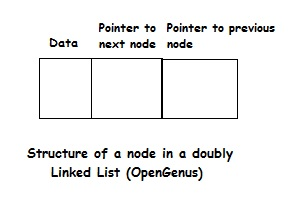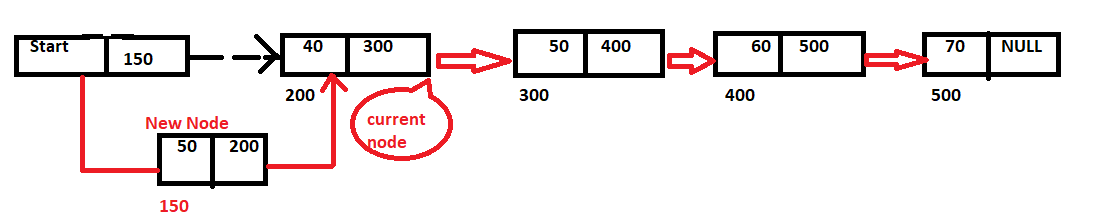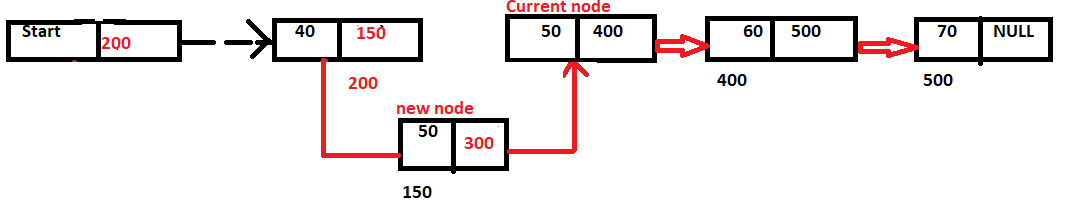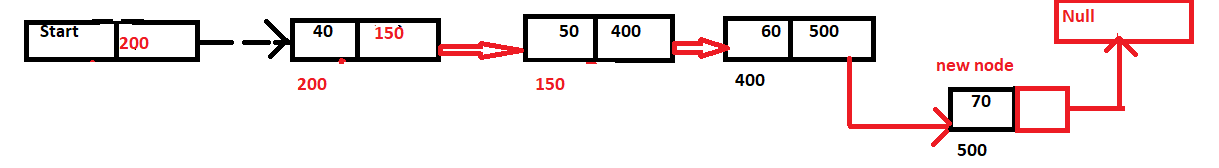×

Search anything:

# Insertion operation in a Linked ListReading time: 15 minutes | Coding time: 20 minutes

Linked List is an efficient data structure to store data. You can learn about the basics of Linked List data structure in this wonderful post. In this session, we will explore the insertion operation in a Linked List.

As we discussed previously, any data structure that stores data has three basic operations:

• Insertion: To insert data into the data structure
• Deletion: To delete data from the data structure
• Search: To search for a data in the data structure

In this leason, we will explore the insertion operation in a Linked List in depth.

The structure of a node in a Linked List is as follows:The structure of a node in a doubly Linked List is as follows:The variants of insertion operation that can be performed are:

• Inserting a node at front of the linked list
• Inserting a node at a particular location in a Linked List
• Inserting a node at the end of the Linked List

### How to insert a node at front of a Linked List?

To insert a node, we have to redirect the next pointer of the previous node to point to the new node instead of the current one and the next pointer of the new node must point to the original next node.

To insert a node at the front of the Linked List, the head pointer should point to the new node and the next pointer of the new node must point to the previous first node.### Pseudocode of inserting a node at the front of a Linked List

``````
{
final Node<E> front = first;
final Node<E> newNode = new Node<>(null, e, front);
first = newNode;
if( front == null)
last = newNode;
else
front.prev = newNode;
++ size;
}
``````

### How to insert a node at a particular location of a Linked List?

To insert a node at a particular location, we have to redirect the next pointer of the previous node to point to the new node instead of the current one and the next pointer of the new node must point to the original next node.### Pseudocode of inserting a node at a particular location of a Linked List

``````
private void LinkBefore(E e, Node<E> succ)
{
Node<E> before = succ.prev;
Node<E> newNode = new Node<E>(before, e, succ);
succ.prev = newNode;
if(before == null)
{
first = newNode;
}
else
{
before.next = newNode;
}
++ size;
}
``````

### How to insert a node at the end of the Linked List?

To insert a node, we have to redirect the next pointer of the previous node to point to the new node instead of the current one and the next pointer of the new node must point to the original next node.

To insert a node at the end of the Linked List, the next pointer of the last node should point to the new node and the next pointer of the new node must point to null.### Pseudocode of inserting a node at the end of a Linked List

``````
{
final Node<E> l = last;
final Node<E> newNode = new Node<>(l, e, null);
last = newNode;
if(l == null)
first = newNode;
else
l.next = newNode;
++ size;
}
``````

### Implementations

Implementations of inserting a node in a Linked List is as follows:

• Java

### Java

``````
import java.util.*;
{
private static class Node<E>
{
E item;
Node<E> next;
Node<E> prev;
Node(Node<E> prev, E element, Node<E> next)
{
this.item = element;
this.prev = prev;
this.next = next;
}
}
transient int size = 0;
transient Node<E> first;
transient Node<E> last;
// Creates an empty list
// Return Node at index "index" O(N) time
public Node<E> node( int index)
{
if(index < (size >> 1))
{
Node<E> x = first;
for(int i=0;i<index; ++i)
x = x.next;
return x;
}
else
{
Node<E> x = last;
for(int i=size-1; i>index; --i)
x = x.prev;
return x;
}
}
// Print all elements in the LinkedList
public void printList()
{
Node<E> x = first;
for(int i=0;i<size; ++i)
{
System.out.println(x.item);
x = x.next;
}
}
{
final Node<E> front = first;
final Node<E> newNode = new Node<>(null, e, front);
first = newNode;
if( front == null)
last = newNode;
else
front.prev = newNode;
++ size;
}
{
final Node<E> l = last;
final Node<E> newNode = new Node<>(l, e, null);
last = newNode;
if(l == null)
first = newNode;
else
l.next = newNode;
++ size;
}
// Inserts a node before a non null node succ
private void LinkBefore(E e, Node<E> succ)
{
Node<E> before = succ.prev;
Node<E> newNode = new Node<E>(before, e, succ);
succ.prev = newNode;
if(before == null)
{
first = newNode;
}
else
{
before.next = newNode;
}
++ size;
}
{
final Node<E> next = f.next;
first = next;
final E element = f.item;
f.item = null;
f.next = null;
if(next == null)
last = null;
else
next.prev = null;
-- size;
return element;
}
{
final E element = l.item;
final Node<E> newLast = l.prev;
l.prev = null;
l.item = null;
last = newLast;
if(newLast == null)
first = null;
else
newLast.next = null;
-- size;
return element;
}
{
final E element = n.item;
final Node<E> before = n.prev;
final Node<E> next = n.next;
if( before == null )
else if(next == null)
else
{
n.item = null;
n.next = null;
n.prev = null;
before.next = next;
next.prev = before;
-- size;
}
return element;
}
{
}
{
}
{
}
}
{
public static void main()
{
Node<Integer> nn = ll.node(3);
ll.printList();
}
}
``````

### Complexity

• Worst case time complexity: `Θ(1)`
• Average case time complexity: `Θ(1)`
• Best case time complexity: `Θ(1)`
• Space complexity: `Θ(1)`#### OpenGenus Tech Review Team

The official account of OpenGenus's Technical Review Team. This team review all technical articles and incorporates peer feedback. The team consist of experts in the leading domains of Computing.

Improved & Reviewed by:

Insertion operation in a Linked List